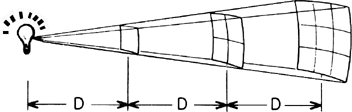# inverse-square law

Also found in: Dictionary, Thesaurus, Medical, Legal, Wikipedia.

## inverse-square law

[′in‚vərs ¦skwer ‚lȯ]
(physics)
Any law in which a physical quantity varies with distance from a source inversely as the square of that distance.

## inverse-square lawinverse-square law
A law which applies to a light source (or to a sound source) that is in a space far away from any reflecting surface: the intensity at a point, as measured on a surface which is perpendicular to a line drawn between the point and the source, varies inversely with the square of the distance between the point and the source. (For sound waves, this decrease in intensity is equivalent to a drop in sound-pressure level of 6 dB for each doubling of distance from the source.)
References in periodicals archive ?
If Hooke is remembered at all, it's for actions during his prime years, when he became a controversial figure for publicly claiming credit for Isaac Newton's inverse-square law of gravity.
Another supporting argument is that the small and stealthy UCAV is survivable enough to get closer to the threat emitter, allowing the inverse-square law to work to an advantage.
Recognition of the laws of motion and of the inverse-square law of universal gravitation were strongly linked with each other, since motions of planets and comets provide the clearest test for any theory of motion.
An interesting example is the borrowing of Newton's inverse-square law by international trade theorists in economics.
If it is as fast, one would expect inverse-square law attenuation of the psi signal.
The inverse-square law of sound intensity decrease with distance would apply: every doubling of distance from the sound source reduces the sound intensity by 6 dB.
Testing the inverse-square law of gravity in boreholes at the Nevada Test Site.

Site: Follow: Share:
Open / Close